blog

# Put Option Payoff Graph

#### Options Trading 101 - The Ultimate Beginners Guide To Options

As Seen On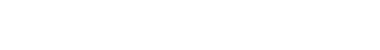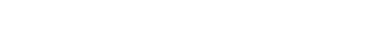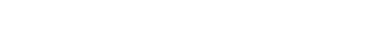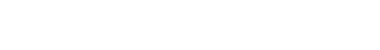by Gavin in BlogToday we’re going to look at the long put option payoff graph. This is designed for beginners so they can develop a basic understanding how to read the payoff graphs.

We’ll look at:

• The payoff graph at expiration and interim dates
• How to calculate the breakeven price
• How time decay will impact the payoff graph
• How volatility will impact the payoff graph

Contents

## Put Option Basics

A long put is a bearish strategy that is employed with limited risk and almost unlimited gain potential..

A put option is a contract between a buyer and seller.

The contract will be for the right to sell a certain stock at a certain price, up until a certain date (called the expiration date).

What this means is that up until the contract expires, the buyer of a put has the right to sell the stock at the agreed price.

## Put Option Payoff Graph

Understanding payoff graphs (or diagrams as they are sometimes referred) is absolutely essential for option traders.

A payoff graph will show the option position’s total profit or loss (Y-axis) depending on the underlying price (x-axis).

Here is an example: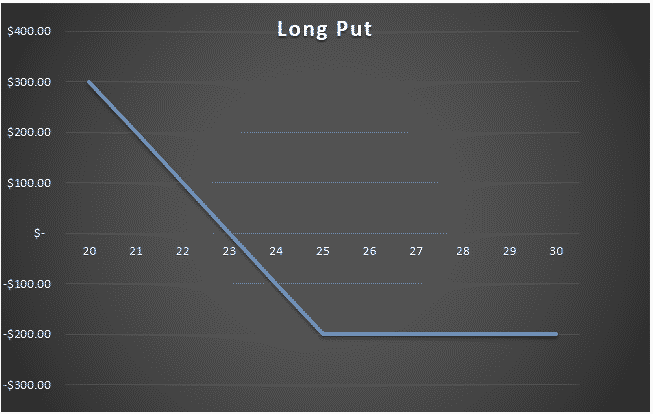What we are looking at here is the payoff graph for a long put option strategy.

In this example the trader has bought a 25 strike put for \$2 per contract (or \$200 for a standard option contract representing 100 shares).

The premium paid of \$200 is the most the trade can lose. That occurs at an underlying stock price of \$25 and above.

Above the strike price of 25, the line rises as the stock price falls.

The lines crosses into profit at a certain point when the stock price falls below the breakeven point.

The breakeven point is simply the strike price minus the premium paid. In this case it would be: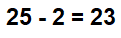If the premium paid had been slightly higher at \$2.20, then the breakeven price would be \$22.80.

## Different Scenarios At Expiration

Four different scenarios can occur at expiration for a long call trade.

1. Underlying stock price is above the strike price

If the underlying stock price is above the strike price at expiration, the put option will expire worthless. The loss for the trade will be equal to the premium paid and no more.

In our example, this would be \$2 per contract or \$200 in total.

Think about it this way, if the stock is trading at \$30 at expiry, it makes no sense to exercise the option to sell the stock at \$25.

The good thing about a long put is that the loss is limited to the premium paid, so it doesn’t matter how low the stock goes, the most the trader can lose is the \$200 in this example.

1. Underlying stock price is equal to the strike price

The stock price ending right at the strike price at expiration would be incredibly rare, but it could happen.

In this case it also doesn’t make sense to exercise the option because the trader could just sell the stock in the market for the same price without having to pay the assignment fees.

1. Underlying stock price is below the strike price but above the breakeven price

If the stock is below the strike price at expiration, the trader may think that’s great, but unless it gets below the breakeven price, it will not end in a profit.

For example, let’s say the stock ends at \$24. The 25 strike put option has \$1 of value left and can either be sold in the market or exercised.

But, the \$1 of value is less than the \$2 paid, so in this scenario, even though the put option has some value at expiration, the trader has lost \$1 per contract.

1. Underlying stock price is lower than the breakeven price

This is the ideal scenario that the trader was hoping for. Ideally the stock would be a long way below the breakeven price.

The trader can either exercise the option, or sell it in the market for a profit.

Let’s assume the stock finished at \$21, the 25 strike put option will have a value of \$4.

The profit to the trader is \$4 less the \$2 paid, for a total profit of \$2.

If the trader chooses to exercise the put option, they will sell short (unless they already own it) the stock at \$25 and can immediately buy it back in the market for \$21.

Or, if they don’t want to exercise the option, they can sell the put in the market before expiration for \$6.

## Payoff Graph At Interim Dates

So far, we’ve only considered the payoff graph at expiration. The payoff graph at interim dates will look very different and will be impacted by factors such as time decay and implied volatility.

Calculating the profit and loss at expiration is quite simply, using excel or even in your head if you are half decent at maths.

Calculating profits and losses at interim dates is much more complex and requires more advanced software than just Excel.

Any decent options broker will have the ability to show option profits and losses at interim dates.

The below put option payoff is from Interactive Brokers.

The put option was an SPY 335 strike put purchased for \$11.10 per contract or \$1,110 in total. The breakeven price at expiration is 323.90 (strike price minus the premium paid).

The blue line shows the expiration payoff that you are now familiar with and the purple line shows what is known as a “T+0” line.

T+0 simply means, what does the trade look like as of today?

Notice that the breakeven price as of today is the current SPY price. This makes sense because we just bought the put so wouldn’t expect to have made or lost any money yet.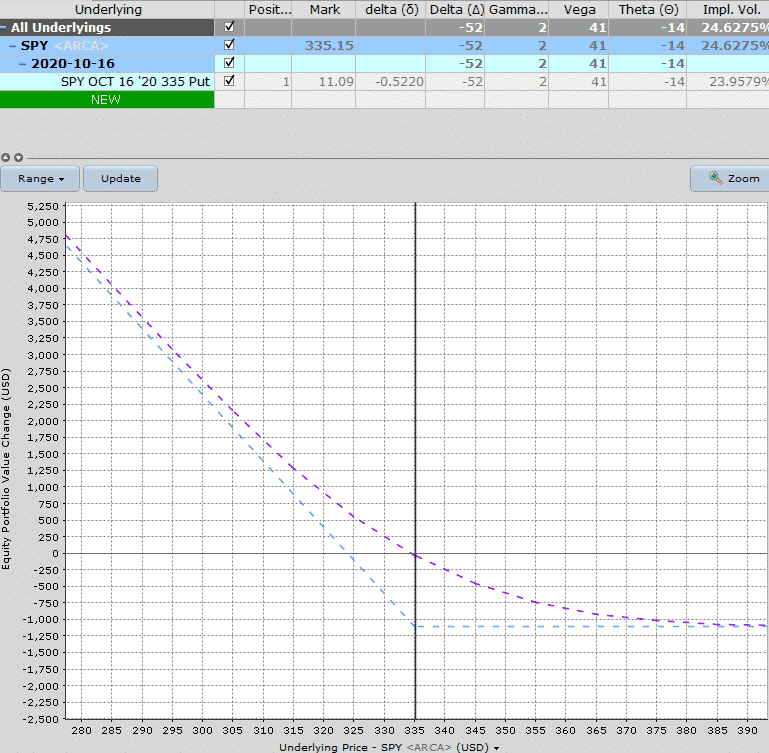Notice also that profits occur much before the expiration breakeven price and above 335, the losses are not the maximum loss.

As time goes by, the interim line slowly moves towards the expiration line.

Here’s how the payoff diagram is estimated to look at one week before expiration.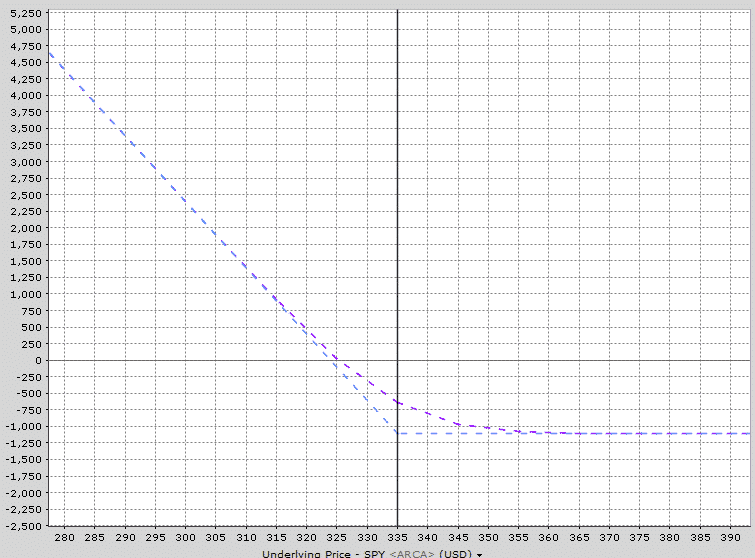See how the purple line is now much closer to the expiration payoff line?

Most brokers will be able to provide this sort of graphical information for you.

I also like to use Option Net Explorer because they can show multiple interim date lines.

Looking at the payoff graph below, notice that there are three interim lines – T+0, T+12 and T+24. This gives the trade a good appreciation of how the trade will progress over time after 12 days and 24 days.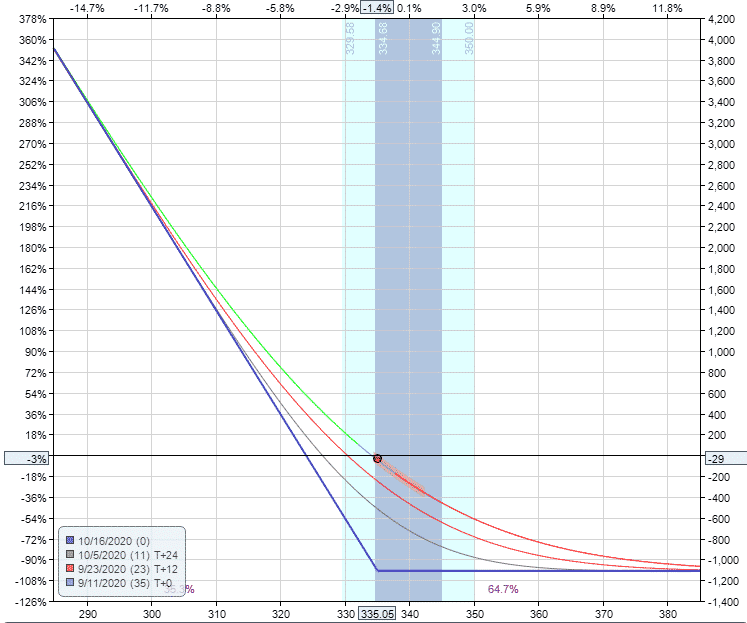## How Changes In Volatility Impact The Payoff Graph

Changes in implied volatility will not have any impact on the payoff graph at expiration, but it will impact the interim dates.

A long put strategy has positive vega, meaning that it will benefit from an increase in implied volatility after placing the trade.

This SPY trade example has vega of +41 which means that for every 1% rise in implied volatility, the trade will make \$41 with all other factors being equal.

The opposite is true if implied volatility drops.

Let’s take a look at how a 20% rise in volatility impacts the interim lines.

Notice that the expiration line hasn’t changed at all, but the interim lines have all moved higher.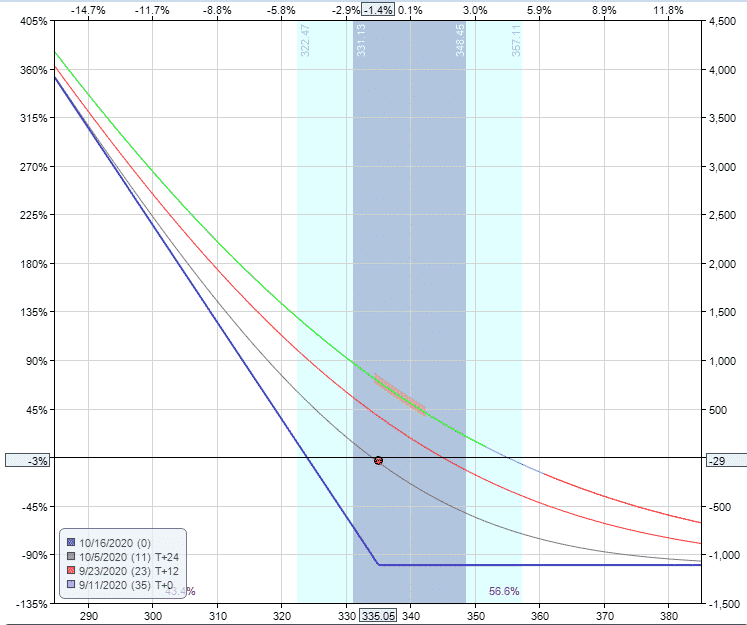Now, let’s see how the diagram changes assuming a 20% drop in volatility. All the interim lines have dropped significantly.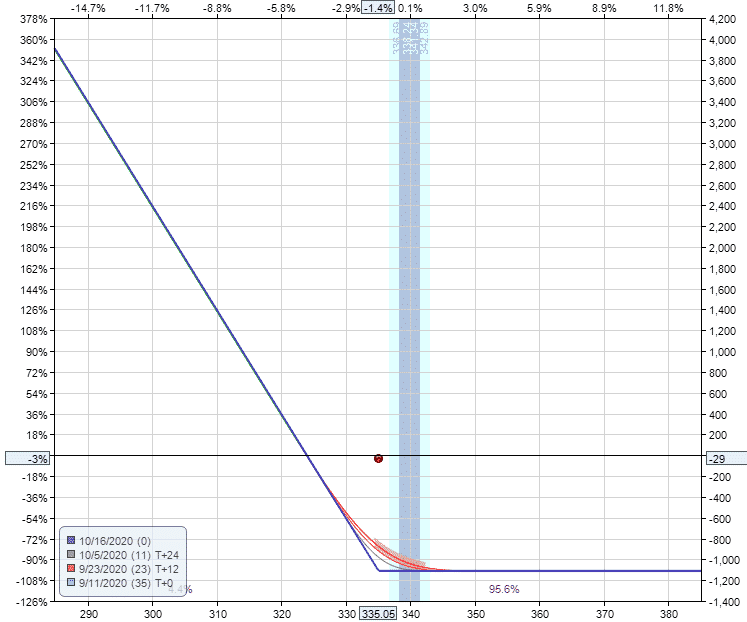With a long put strategy, delta is the main driver of the trade, but we can clearly see that big changes in implied volatility will also affect the trade.

## Summary

You should now be intimately familiar with the put option payoff graph. Let’s summarize some key points.

• Long put options are a bearish strategy with limited risk and unlimited upside
• The maximum loss is equal to the premium paid for the long put
• The breakeven price is equal to the strike price minus the premium paid
• The position will lose value as expiration approaches due to time decay
• As a positive vega trade, increases in implied volatility will help the trade, but only at interim dates

If you’re still having trouble or want to learn more about these concepts, you can check out this 12,000 word beginners guide to options.